Question

# Determine the internal normal force, shear force, and the moment at points C and D.

Determine the internal normal force, shear force, and the moment at points C and D.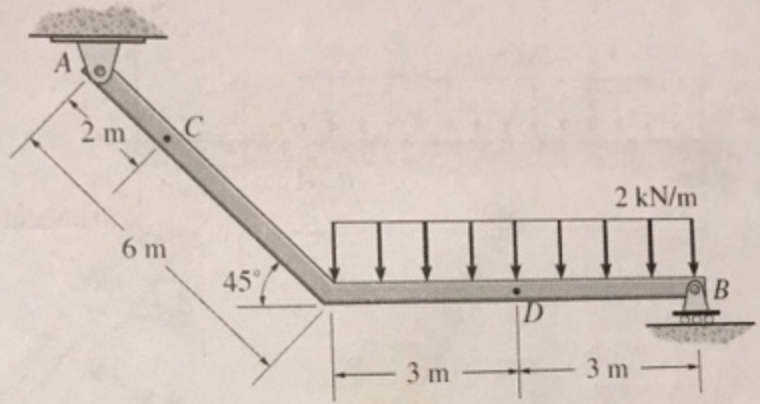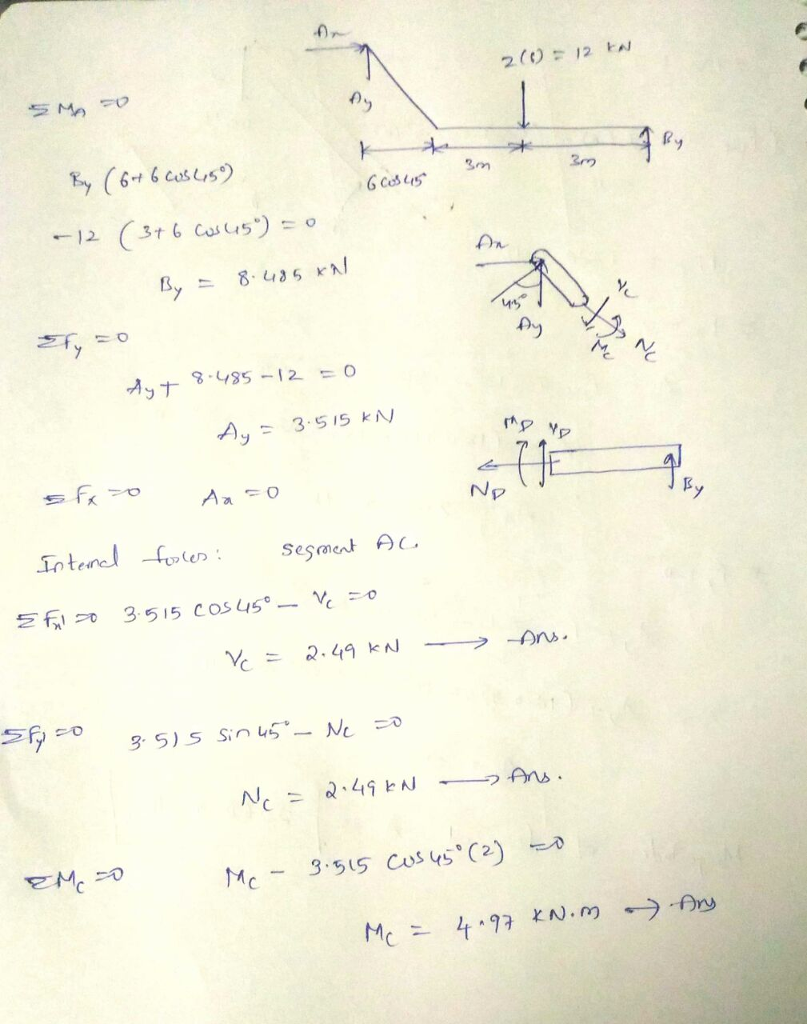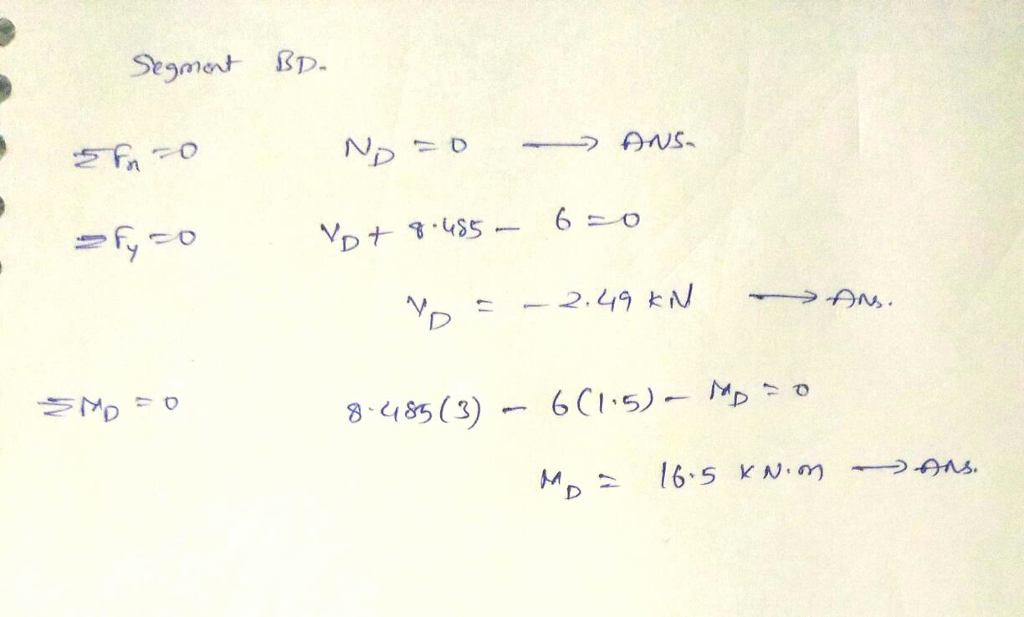#### Earn Coins

Coins can be redeemed for fabulous gifts.

Similar Homework Help Questions
• ### Determine the internal normal force, shear force, and moment at points C and D in the...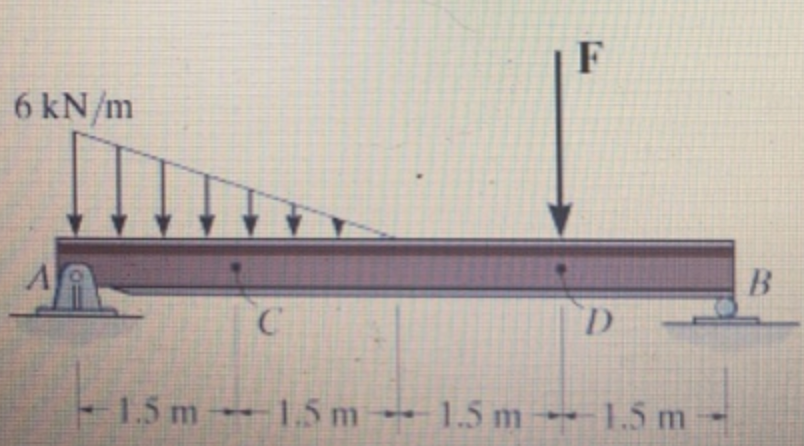Determine the internal normal force, shear force, and moment at points C and D in the simply supported beam. Point D is located just to the left of the F = 15 kN concentrated load. (Figure 1)

• ### Determine the internal normal force, internal shear force, and internal moment at points D and E of the frame.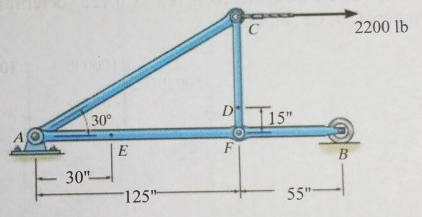Determine the internal normal force, internal shear force, and internal moment at points D and E of the frame.

• ### Determine the internal normal force, shear force, and moment at point D.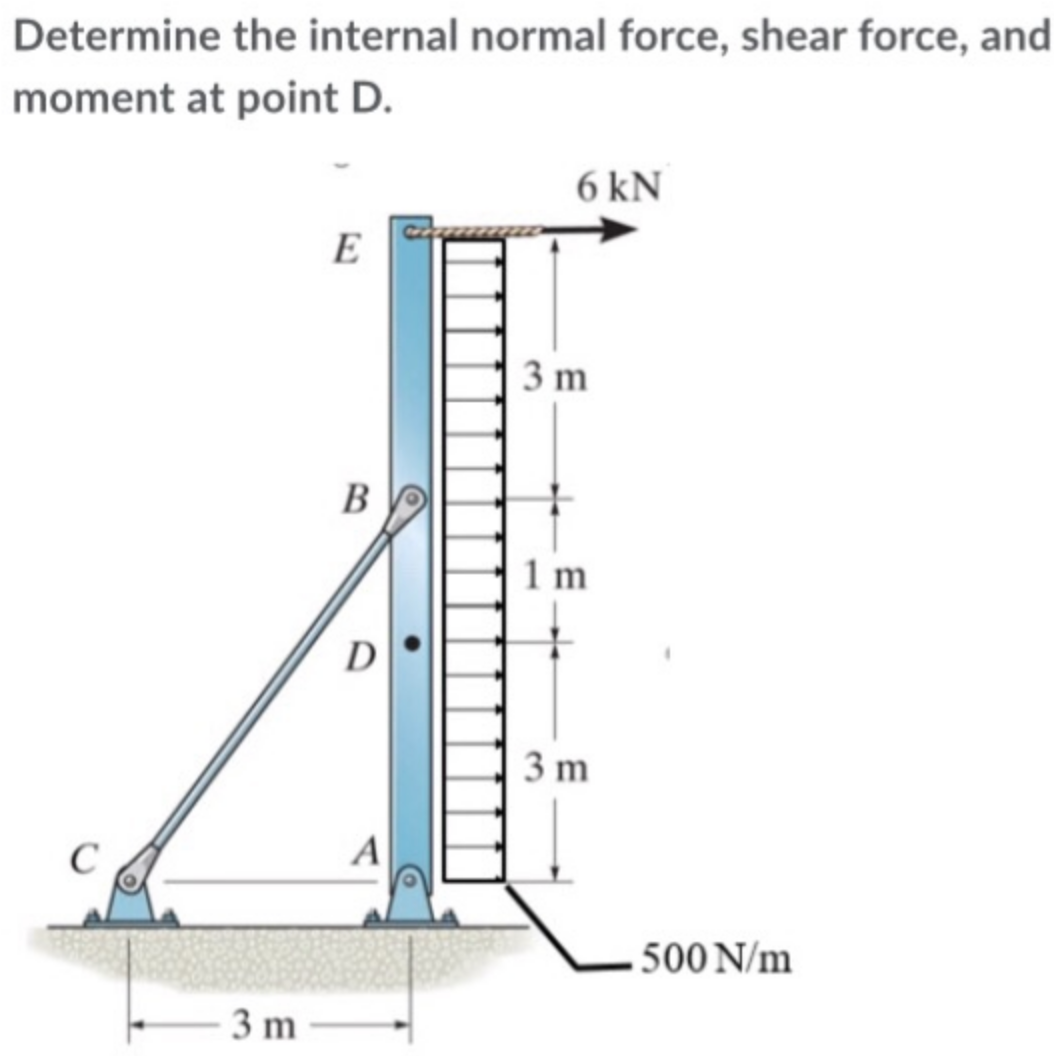Determine the internal normal force, shear force, and moment at point D.

• ### Determine the internal normal force, shear force, and moment at points and D in the simply supported beam.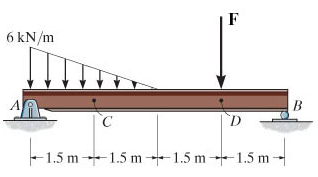Determine the internal normal force, shear force, and moment at points and D in the simply supported beam. Point Dis located just to the left of the F = 14 kN concentrated load.(Figure 1)

• ### Determine the internal normal force, shear force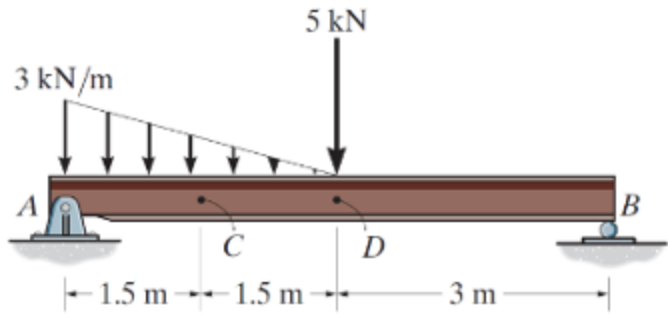Determine the internal normal force, shear force, and moment at points C and D in the simply supported beam using method of sections. Point D is A located just to the left of the 5-kN force. Also, draw shear and moment diagrams using the graphical method.

• ### Determine the internal normal force, shear force, and bending moment at point C in the beam.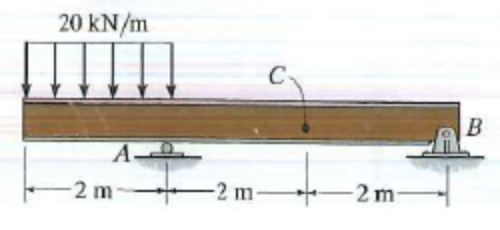Determine the internal normal force, shear force, and bending moment at point C in the beam.

• ### Problem 3. (38% ) Determine the internal normal force, shear force, and moment at points A...Problem 3. (38% ) Determine the internal normal force, shear force, and moment at points A and B in the frame. The supports at C and D are both pins. Present a F.B.D. for each analysis performed. KN 4(3)-12KN 4 kN/m Cak Cy 2 m 12, KN A 1.5 1-5m 1m- 1 m m. Crt (タニP× 1Z k Thar EMC-0 -12 (1.5) P (2) =o Bh-C=O Dry. -9KN FrD A-(121-6 EMc-o (e.6) M Problem 3. (38% ) Determine the internal...

• ### Calculate the internal normal force, internal shear force, and internal bending moment at a location 0.75m...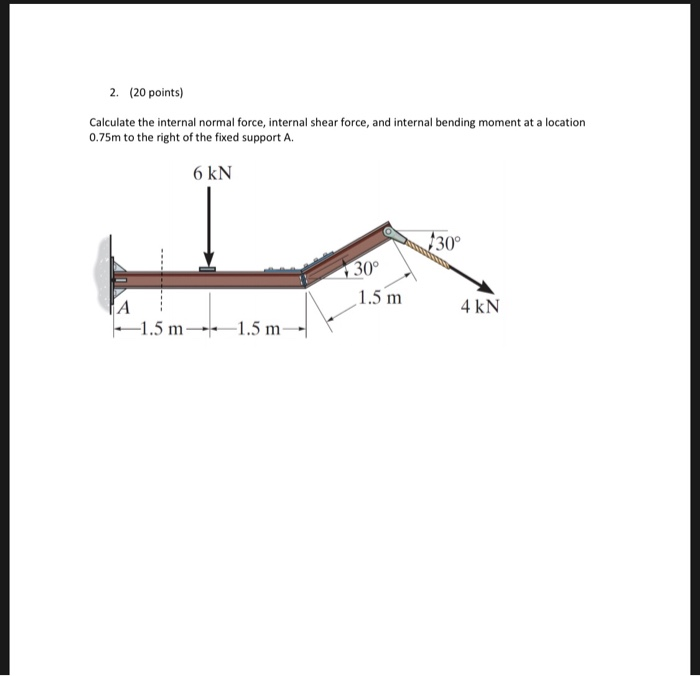Calculate the internal normal force, internal shear force, and internal bending moment at a location 0.75m to the right of the fixed support A. 2. (20 points) Calculate the internal normal force, internal shear force, and internal bending moment at a location 0.75m to the right of the fixed support A 6 kN 30° 30° 1.5 m 4 kN 1.5 m1.5 m

• ### Type or paste question here P3: Determine the internal normal force, shear force, and bending moment...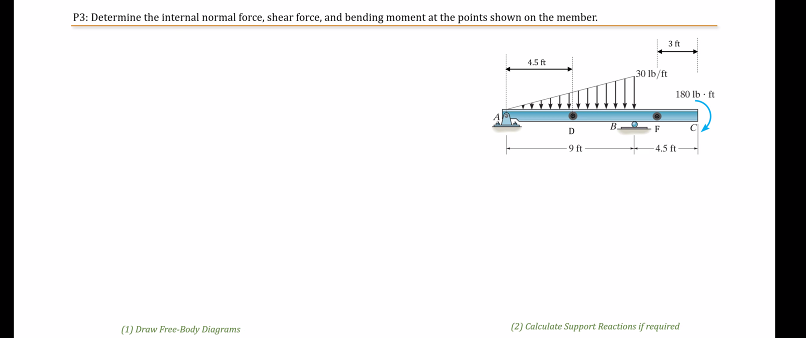Type or paste question here P3: Determine the internal normal force, shear force, and bending moment at the points shown on the member. 3 4.5 ft 30 lb/ft 180 lb.it DBF C -9ft — -—4.5ft- (1) Draw Free-Body Diagrams (2) Calculate Support Reactions if required

• ### determine the normal force,shear force and bending moment at C of the beam in the figure...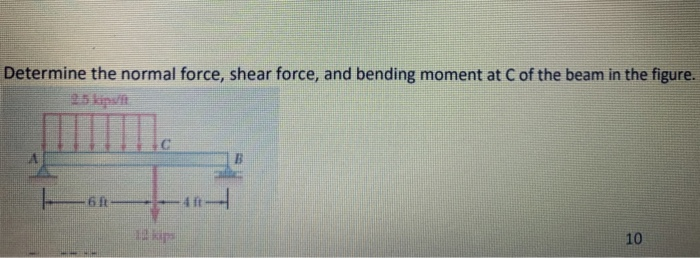determine the normal force,shear force and bending moment at C of the beam in the figure Determine the normal force, shear force, and bending moment at C of the beam in the figure. 25 kips 10